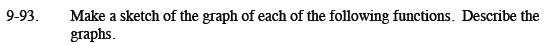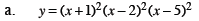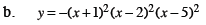Home > CCA2 > Chapter 9 > Lesson 9.3.2 > Problem9-93

9-93.
1. Make a sketch of the graph of each of the following functions. Describe the graphs. Homework Help ✎

1. y = (x + 1)2(x − 2)2(x − 5)2

2. y = −(x + 1)2(x − 2)2(x − 5)2Identify the y-intercept and all of the x-intercepts.

What is the degree of the polynomial?
How does this relate to the end behavior of the graph?

Any factor that is 'squared' creates a double root.
What do double roots look like on a graph?This graph is almost the same as the graph in part (a).
How is it different?